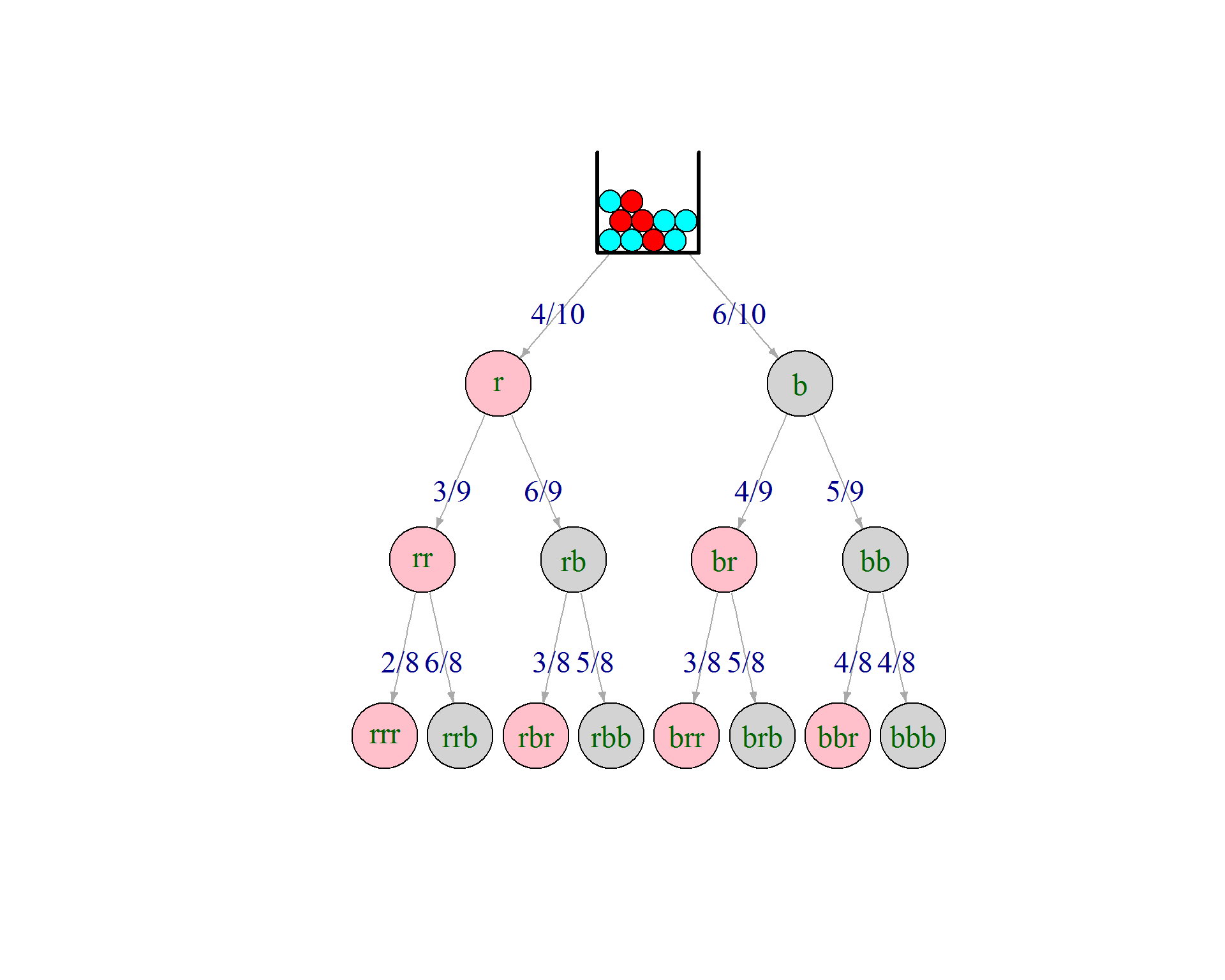## Alternative to the Hypergeometric Formula

The Hypergeometric Distribution is usually explained via an urn analogy and formulated as the ratio of “favorable outcomes” to all possible outcomes:

$\displaystyle \boxed{P(x=a;N,A,n,a) = \frac{{A \choose a} \cdot {N-A \choose n-a} }{{N \choose n}}}$

where $$N$$ is the total number of balls in, $$A$$ the number of “red” balls in the urn, and $$n$$ the sample size. The question answered by the above expression is the probability of finding $$x=a$$ red balls in the sample.

However, a different – equally worthy – viewpoint is that of a tree with conditional probabilities.

Here is an example for $$N=10, A=4, n=3$$So there are 3 leafs with exactly one red ball in the sample. Following the tree we multiply the conditional probabilities of the tree egdes to get to the “and” probability of the leafs. It should be clear that all three probabilites are identical – but for the order of multiplication:

$P (\mbox{one red}) = 3 \cdot P_{leaf} = 3 \cdot \frac{4}{10} \cdot \frac{6}{9} \cdot \frac{5}{8} = 3 \cdot \frac{4 \cdot 6 \cdot 5}{10 \cdot 9 \cdot 8}$

### Alternative formula

How many leafs contain exactly one red ball? Exactly $${n \choose a} = {3 \choose 1} = 3$$. The probability for the precise event “a red balls followed by n-a blue balls”is:

$\frac{A}{N} \cdot \frac{A-1}{N-1} \cdots \frac{A-a+1}{N-a+1} \cdot \frac{N-A}{N-a} \cdot \frac{N-A-1}{N-a-1} \cdots \frac{N-A-(n-a-1)}{N-a-(n-a-1)}$
The last denominator is simply $$N-n+1$$, i.e. the full denominator is $$_NV_n=N!/(N-n)!$$

The left numerator is simply $$_AV_n = A!/(A-a)!$$ in analogy the right numerator $$_{N-A}V_{n-a} = (N-A)!/(N-A-(n-a))!$$ So, all in all ${n \choose a} \cdot \frac{_AV_n \cdot _{N-A}V_{n-a}}{_NV_n} = {n \choose a} \cdot \frac{A! \cdot (N-A)! \cdot (N-n)!}{(A-a)! \cdot (N-A-(n-a))! \cdot N!}$

$= {n \choose a} \cdot \frac{\frac{A!}{(A-a)!} \cdot \frac{(N-A)!}{(N-A-(n-a))!} }{\frac{N!}{(N-n)!}} = \frac{{A \choose a} \cdot {N-A \choose n-a} }{{N \choose n}}$

which leaves us with $\displaystyle \boxed{P(x=a;N,A,n,a) = {n \choose a} \cdot \frac{_AV_n \cdot _{N-A}V_{n-a}}{_NV_n} }$